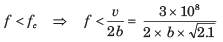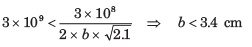Courses

# Test: Waveguides

## 20 Questions MCQ Test RRB JE for Electrical Engineering | Test: Waveguides

Description
This mock test of Test: Waveguides for Electronics and Communication Engineering (ECE) helps you for every Electronics and Communication Engineering (ECE) entrance exam. This contains 20 Multiple Choice Questions for Electronics and Communication Engineering (ECE) Test: Waveguides (mcq) to study with solutions a complete question bank. The solved questions answers in this Test: Waveguides quiz give you a good mix of easy questions and tough questions. Electronics and Communication Engineering (ECE) students definitely take this Test: Waveguides exercise for a better result in the exam. You can find other Test: Waveguides extra questions, long questions & short questions for Electronics and Communication Engineering (ECE) on EduRev as well by searching above.
QUESTION: 1

### Consider a 150 m long air-filled hollow rectangular wave guide with cutoff frequency 6.5 GHz. If a shortpulse of 7.2 GHz is introduced into the input end of theguide, the time taken by the pulse to return the inputend is

Solution: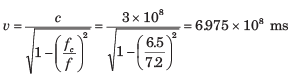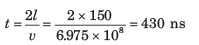QUESTION: 2

### In an air-filled rectangular waveguide the cutoff frequencies for TM11 and TE03 modes are both equal to 12 GHz. Que: The dominant mode is

Solution: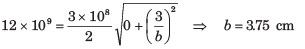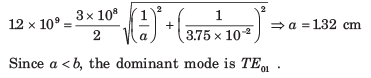QUESTION: 3

### In an air-filled rectangular waveguide the cutoff frequencies for TM11 and TE03 modes are both equal to 12 GHz. Que:At dominant mode the cutoff frequency is

Solution: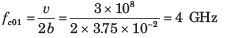QUESTION: 4

For an air-filled rectangular waveguide given that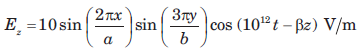If the waveguide has cross-sectional dimension a = 6 cm and b = 3 cm, then the intrinsic impedance of this mode is

Solution: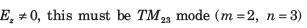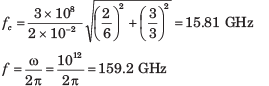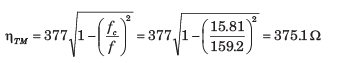QUESTION: 5

In an air-filled waveguide, a TE mode operating at 6 GHz has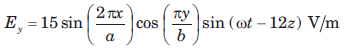Que: The cutoff frequency is

Solution: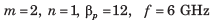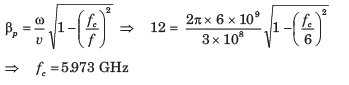QUESTION: 6

In an air-filled waveguide, a TE mode operating at 6 GHz hasQue: The intrinsic impedance is

Solution: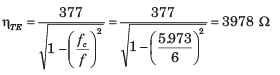QUESTION: 7

Consider an air-filled rectangular wave guide with a = 2.286 cm and b = 1.016 cm. The y-component of the TE mode is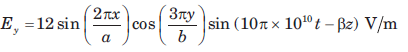Que: The propagation constant γ is

Solution:

m = 2, n = 3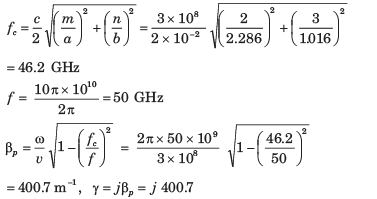QUESTION: 8

Consider an air-filled rectangular wave guide with a = 2.286 cm and b = 1.016 cm. The y-component of the TE mode isQue: The intrinsic impedance is

Solution: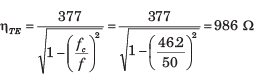QUESTION: 9

Consider a air-filled waveguide operating in the TE12 mode at a frequency 20% higher than the cutoff frequency.

Que: The phase velocity is

Solution:

v = c, f = 1.2 fc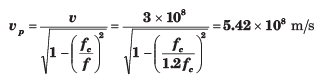QUESTION: 10

Consider a air-filled waveguide operating in the TE12 mode at a frequency 20% higher than the cutoff frequency.

Que: The group velocity is

Solution: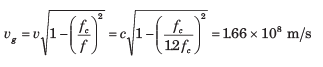QUESTION: 11

A rectangular waveguide is filled with a polyethylene ( εr = 2.25 ) and operates at 24 GHz. The cutoff frequency of a certain mode is 16 GHz. The intrinsic impedance of this mode is

Solution: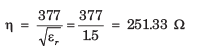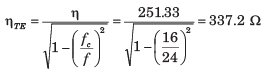QUESTION: 12

own in fig. It has dielectric discontinuity as shown in fig. If the guide operate at 8 GHz in the dominant mode, the standing wave ratio is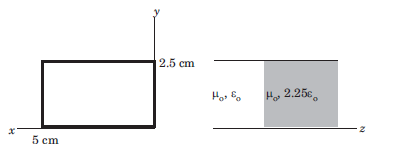Solution:

Since a > b, the dominant mode is TE10 .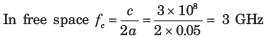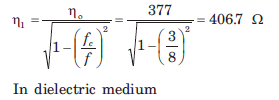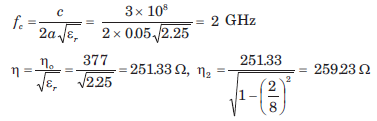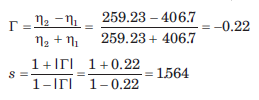QUESTION: 13

Consider the rectangular cavity as shown in fig.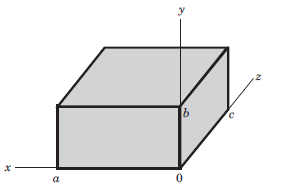Que: If a < b < c, the dominant mode is

Solution: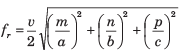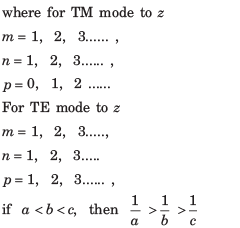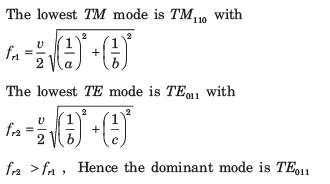QUESTION: 14

Consider the rectangular cavity as shown in fig.Que: If a > b >  c, then the dominant mode is

Solution: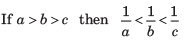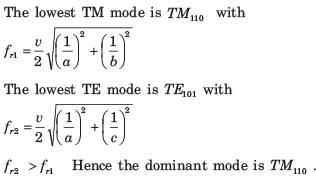QUESTION: 15

Consider the rectangular cavity as shown in fig.Que: If a = c > b, then the dominant mode is

Solution: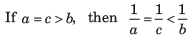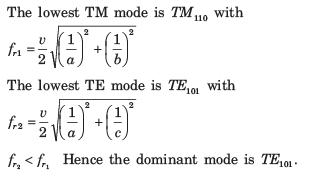QUESTION: 16

The air filled cavity resonator has dimension a = 3 cm, b = 2 cm, c = 4 cm. The resonant frequency for the TM110 mode is

Solution: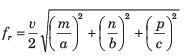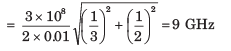QUESTION: 17

A 6 cm x 4 cm rectangular wave guide is filled with dielectric of refractive index 1.25

Que: The range of frequencies over which single mode operation will occur is

Solution: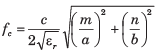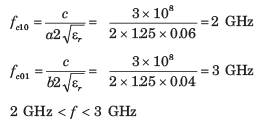QUESTION: 18

A 6 cm x 4 cm rectangular wave guide is filled with dielectric of refractive index 1.25

Que: The range of frequencies, over which guide support both TE10 and TE01 modes and no other, is

Solution: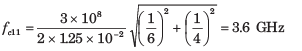3 GHz < f < 3.6 GHz

QUESTION: 19

Two identical rectangular waveguide are joined end to end where a = 2b. One guide is air filled and other is filled with a lossless dielectric of εr. it is found that up to a certain frequency single mode operation can be simultaneously ensured in both guide. For this frequency range, the maximum allowable value of εr is

Solution: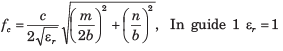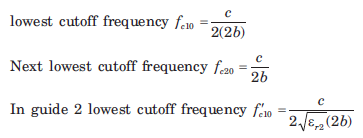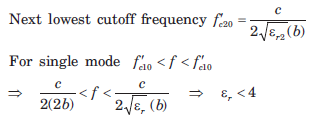QUESTION: 20

A parallel-plate guide operates in the TEM mode only over the frequency range 0 < f < 3 GHz. The dielectric between the plates is teflon (εr = 2.1) .The maximum allowable plate separation b is

Solution: